# Better Data Tables with VBA

On this webpage I deal with a number of serious problems inherent with data tables using simple VBA programs. I demonstrate that relatively simple and short VBA code can be used as an alternative to data tables that the problems.  The VBA alternative to data tables can be made with a couple of lines of code using a FOR and NEXT loop along with the CELLS tool. You can make the code very flexible so that it can start on different rows and different columns. You can also make the code flexible so you can use alternative range names for inputs and outputs. The VBA to make data tables is a good way work through the fundamental ideas of creating VBA programs.

When you use VBA code instead of data tables you can fix many of the problems. You can illustrate the scenario analysis with a graph because you don’t have to fill in the blank with a number.  If you have a big database file while your little file with a data table is open (even with the calculation method adjusted).  This will slow things down a lot.  Say you do not want to use code numbers but you want to put your sensitivity analysis in another sheet. You will be stuck.  If you have a goal seek and would like to make your sensitivity analysis work with the goal seek you will also be frustrated.

VBA code can fix problem with data tables. These problems involve writing a macro that should take two minutes:

• Inputs for the data table must be in same sheet as the data table itself.  This is fixed by using range names and changing the inputs with the CELLS function.
• Data tables slow you down when save and open even if using automatic except data tables calculation option is selected
• When you press the F9 to re-calculate, you can mess things up even if you have a large data table
• Because of the restricted formatting when you make a data table, you cannot easily make a graph with the F11 key.
• You cannot use the goal seek with a data table where excel would somehow magically know to run the goal seek function every time the sensitivity tables are run.
• It can be a pain to put the input variables in different sheets when do not use code numbers.
• Data tables cannot be used with copy and paste macros (they can be used with the UDF method).

## Example with VBA and Data Tables

I have made a simple file that demonstrates how to make multiple data tables and solve the problems with data tables in excel. This file includes the VBA code that is demonstrated in the video below and creates four different flexible data tables from the valuation analysis.

I am using the case of building data tables with VBA to demonstrate some VBA principles of FOR/NEXT and using VBA with the INDEX function in excel.  In the exercise, I use a very simple valuation example where we want to investigate the effect of WACC, Terminal Growth, Short-term Growth and the length of the explicit period.  The screenshot below illustrates the inputs.  Note that I put names next to the inputs and I used the SHIFT, CNTL, F3 keystrokes to make named ranges.  I also put the base case values with range names next to the values that are used in the model.  The range names are important because they are used in the VBA code.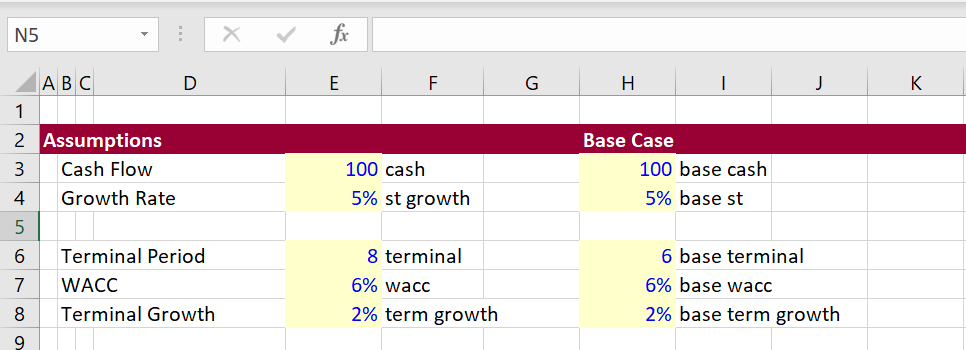The second screenshot demonstrates the model with outputs that will be used in the data tables.  Note that I put a name next to the total value and next to the terminal value which will be outputs of a series of data tables.  The model allows you to use alternative terminal value dates and includes a half-year convention.  I pretend that you want a whole lot of data tables with different explicit periods; different short-term growth rates as well as the WACC and the terminal growth which are in almost every sensitivity table.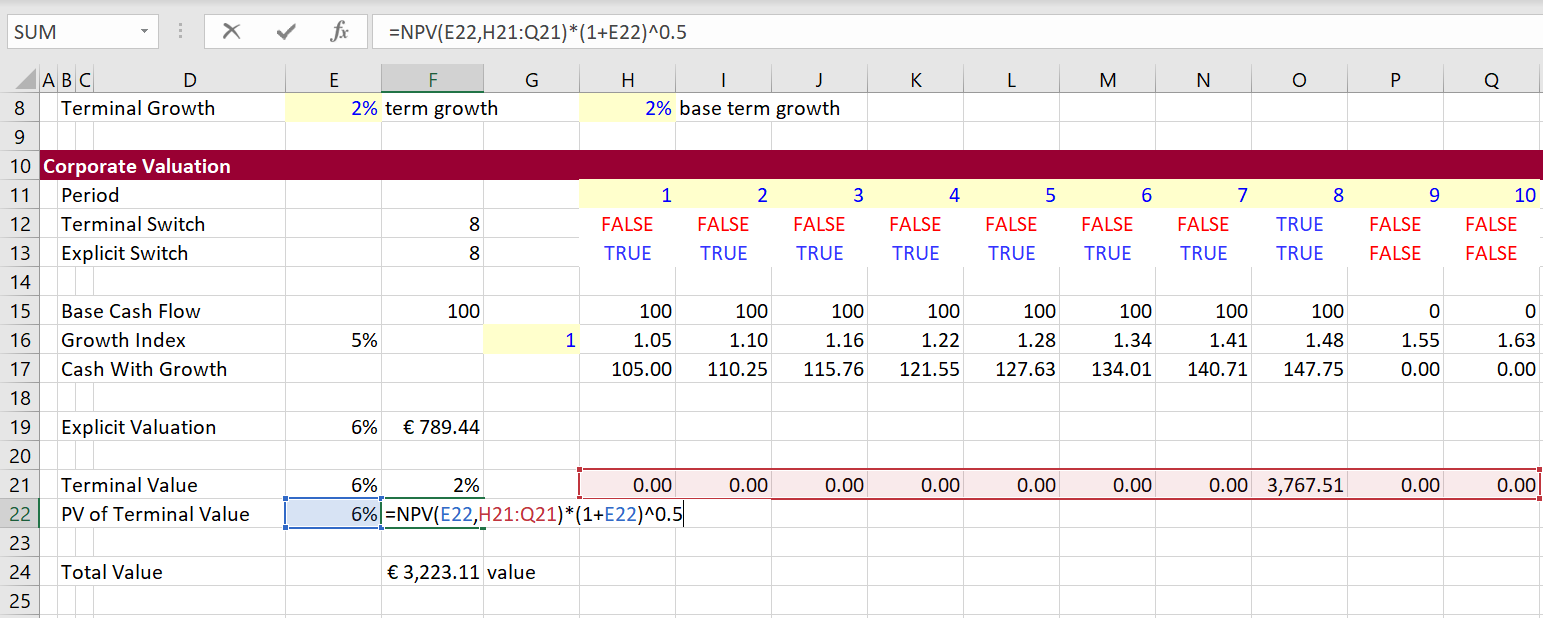Now let’s move to creation of the data tables in a new sheet.  The data tables will not have the irritating thing in the middle that prevents you from making graphs.  The format of the graphs is shown in the screenshot below.  Note that I have chosen different variables for the row and column input.To make the data tables, define the row start, row end, column start and column end.  Then also define the range names that will be used for the row input and column input.  When entering the data, use in INDEX function so that you can choose alternative data tables.  The VBA code will move through the different data tables by changing the code number.  The values that are currently in use are in the left hand side of the table. As the data table 4 is in place, the start row is 35 and the start column is 40 and the output is the terminal value.  You can make as many data tables as you want and this will work with by changing code number that is shown is 4 in the example below.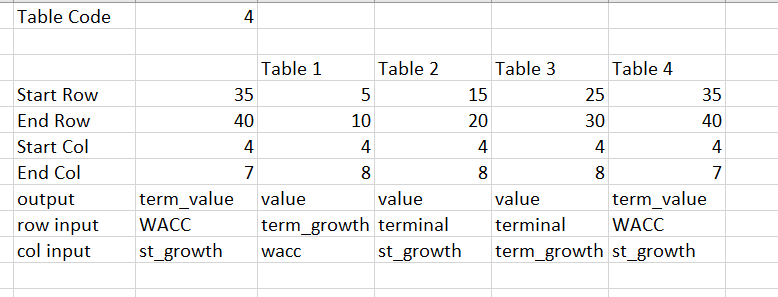Now lets run the data table.  This is done with VBA code that loops through the rows and columns of the data table defined from the range names shown in the screenshot above.  The VBA code is illustrated at the bottom of this explanation.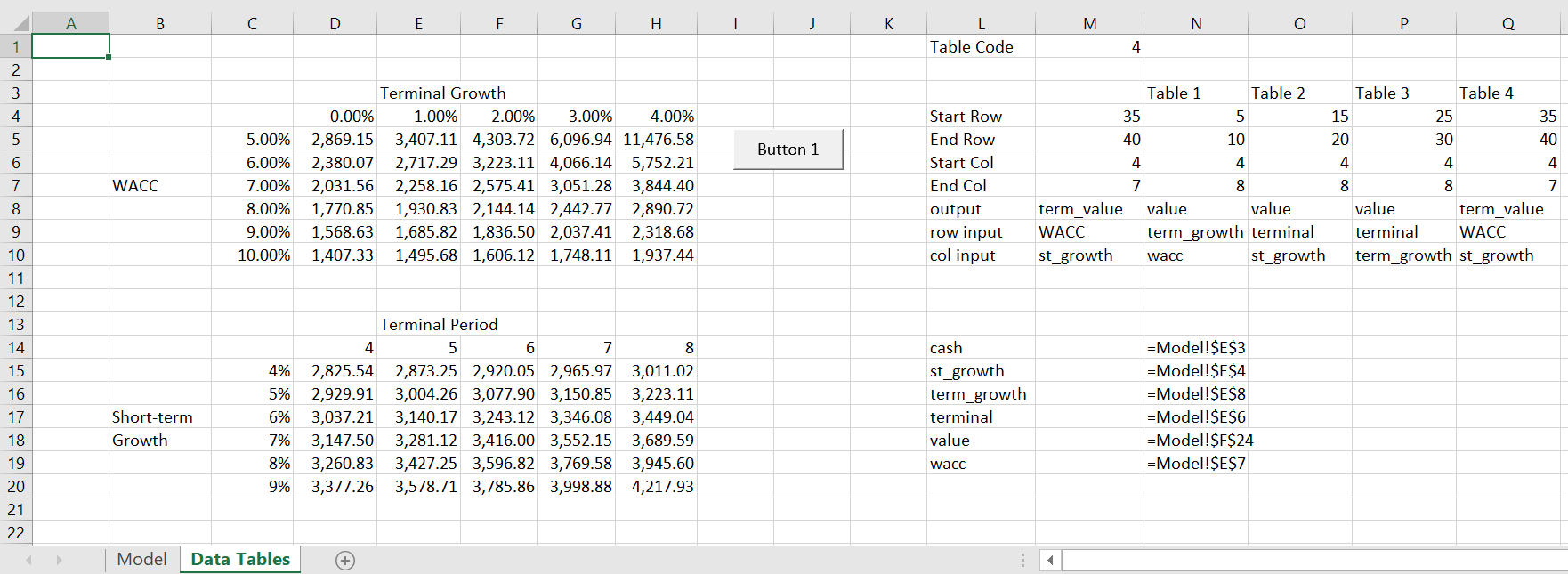The VBA code is shown below.  There are two programs.  The first program creates one data table with FOR/NEXT and the CELLS statements.  You go up one row from the start row to define the series of row scenarios.  You go one to the left to get the column scenarios associated with the column input.  The code includes an error check and at the end of the data table I re-assigned the data to the base case.

.

```Sub table()

'cash
'st_growth
'term_growth
'terminal
'Value
'wacc

For Row = Range("start_row") To Range("end_row")

Range(Range("col_input")) = Cells(Row, Range("start_col") - 1)

For col = Range("start_col") To Range("end_col")
Range(Range("row_input")) = Cells(Range("start_row") - 1, col)

On Error GoTo out_of_loop:
Cells(Row, col) = Range(Range("output"))

back_to_loop:

Next col
Next Row

'
' Reset the values to base case
'

Range("cash") = Range("Base_cash")
Range("st_growth") = Range("Base_st")
Range("term_growth") = Range("Base_terminal")
Range("wacc") = Range("Base_wacc")
Range("term_growth") = Range("base_term_growth")

Exit Sub

out_of_loop:

Resume back_to_loop:

End Sub

.```

The second VBA code demonstrates how to create multiple data tables from this code.  You define the code used in the INDEX excel function.  Then you use a FOR/NEXT loop to change the index code number.

.

```Sub all_tables()

For table_no = 1 To 4

Range("table_code") = table_no
table

Next table_no

End Sub

.```

.

## Graphs with Data Tables made by VBA

One of the things I really hate about data tables made with excel is that you cannot quickly make graphs because of the number at the top left of the table.  With the VBA method you have much more flexibility.  With the data tables created from the code shown in the associated file, you can select the area of the table including the row and column sensitivity variables.  Then you can press F11 or press ALT and F9.  This will create a graph that shows the inter-relationships between the variables.  This is demonstrate in the screenshots below.  The second screenshot shows how you can change the row and column variables by clicking on the select data thing.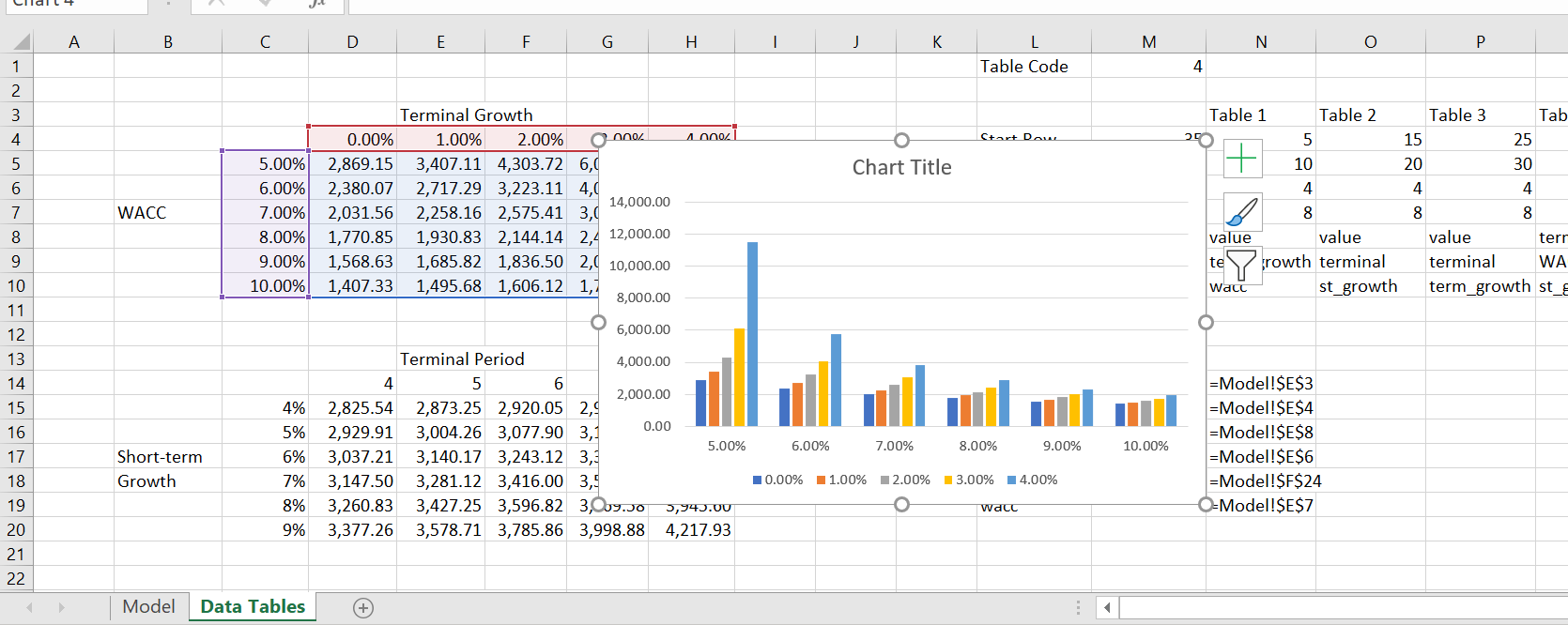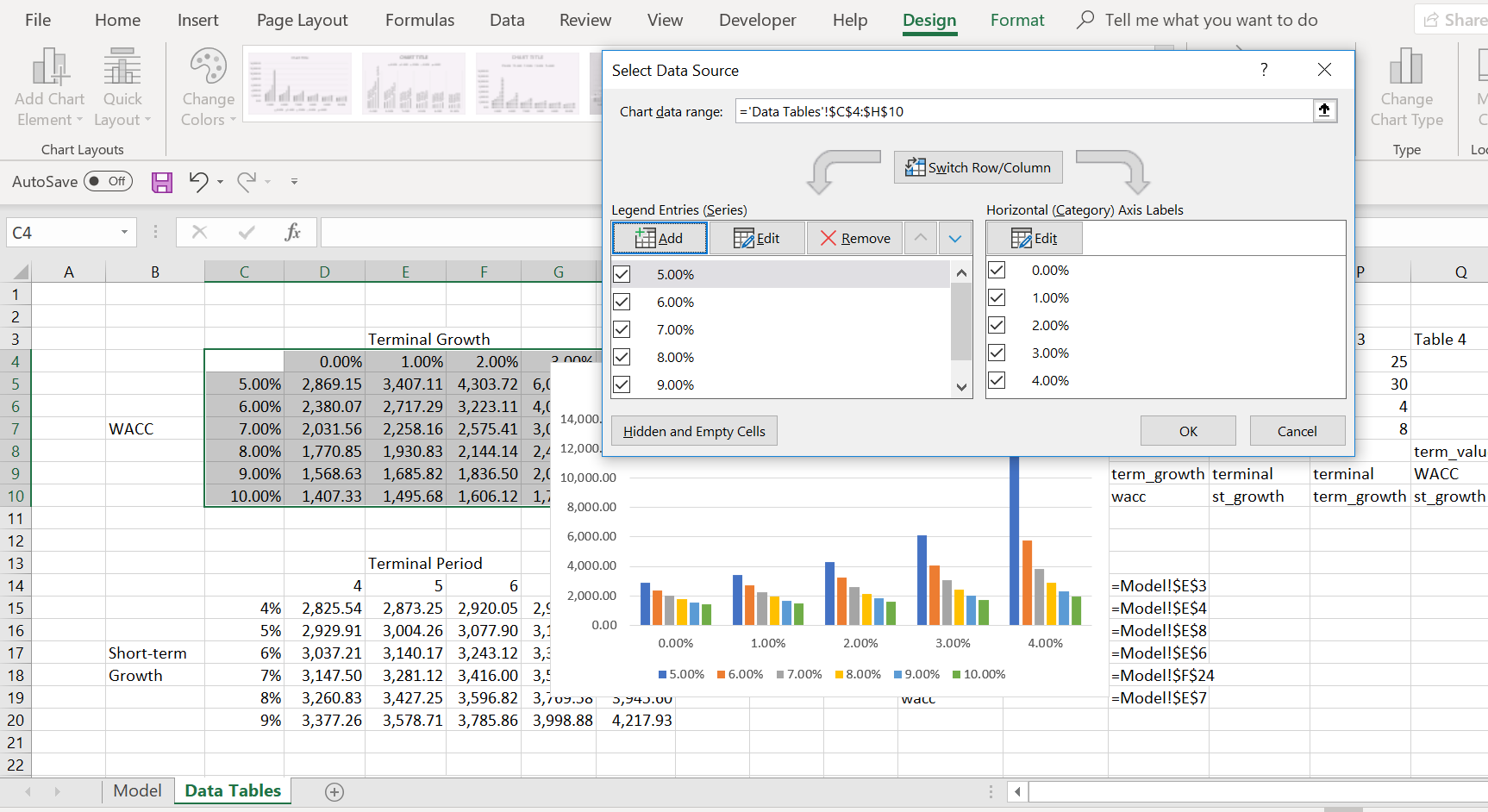.

.

## Speeding up Data Tables by Assigning the Output to a Range Name

Going around rows and columns can take some time.  To speed things up you can assign the output to an array variable.  This is demonstrated in the little test VBA code below.

```.

Sub table()

Dim temp_out(9000, 1) As Single

num = Range("row_end") - Range("row_start") + 1

range_name = "P" & Range("row_start") & ":P" & Range("row_end")

num = 1

For Row = Range("row_start") To Range("row_end")

temp_out(num, 1) = Range("total_cost_for_hour")

num = num + 1

Next Row

Range(range_name) = temp_out

End Sub

.
.```